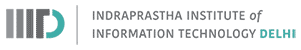## Ontology Quality Assessment

Monsoon 2018
Instructor: Raghava Mutharaju
IIIT-Delhi### Introduction

• How can we evaluate the quality of an ontology?
• The most straightforward criteria is does the ontology answer the competency questions?
• We will discuss some guidelines that can be used to assess ontology quality

### Ontology Quality Assessment Guidelines

1. Logical Criteria
2. Structural and Formal Criteria
3. Accuracy Criteria

### Logical Criteria

• Ontology should not be inconsistent
• This indicates a modelling error
• This is a severe form of global inconsistency
• Weaker form of inconsistency (unsatisfiability) is if a class is empty due to logical constraints
• Horse $\sqsubseteq$ $\neg$ Flies
• FlyingHorse $\sqsubseteq$ Horse $\sqcap$ Flies
• This forces FlyingHorse class to be empty. There cannot be something like ClassAssertion(FlyingHorse pegasus)
• Define a class if it has at least one possible instance. Other ontology is incoherent and might have a modelling flaw
• Ontology should be logically complete
• Ontology should contain as much as information of the domain as possible - at least sufficient to answer all the competency questions
• Check for completeness of each axiom type - SubClassOf, property axioms, class expressions

### Structural and Formal Criteria

• Circular chain of subclass axioms
• Building $\sqsubseteq$ Architecture
• Architecture $\sqsubseteq$ Faculty (weird but assume so)
• Faculty $\sqsubseteq$ University
• University $\sqsubseteq$ Building
• Class rigidity
• A class is rigid if every member of the class cannot cease to be a member without losing existence
• Person class is rigid (a person cannot stop being a person)
• Student class is anti-rigid (a student can stop being a student)
• A rigid class cannot be the subclass of an anti-rigid one

### Accuracy Criteria

• Ontology should model the state of affairs in the real world as faithfully as possible
• Checking this aspect is subjective
• Wherever possible, we can take the help of domain experts in cross verifying the logical axioms in the ontology

### Modelling Suggestions

1. Don't forget disjointness
2. Don't forget property characteristics
3. Don't choose too specific domains or ranges
4. Be careful with quantifiers
5. Don't mistake parts for subclasses
6. Watch the direction of properties
7. Don't confuse class subsumption and class equivalence
8. Don't translate too verbally

#### Disjointness

• Class disjointness axioms are usually forgotten
• But unless specifically stated that classes are disjoint, they are not
• Man $\sqsubseteq$ Human
• Woman $\sqsubseteq$ Human
• Human $\sqsubseteq$ Man $\sqcup$ Woman
• Man(john)
• Woman(mary)
• Is john not a Woman? ($\neg$ Woman(john))
• Add Man and Woman are disjoint (Man $\sqcap$ Woman $\sqsubseteq$ $\bot$)
• Consider siblings in a class hierarchy and if an individual cannot be an instance of both the classes, then declare them as disjoint

#### Role Characteristics

• For each property declared in the ontology consider if the following characteristics apply to it
• transitive
• symmetric
• functional
• inverse functional
• reflexivity
• irreflexivity
• antisymmetry
• property chain
• property disjointness

#### Domain and Range

• For each property declared in the ontology check the usage and verify whether the declared domain and range applies to all the usages
• Multiple ranges (domains) for a property should be interpreted as intersection of all the ranges (domains)
• ObjectPropertyRange(authorOf Textbook)
• ObjectPropertyRange(authorOf Storybook)
• range of authorOf is both Textbook and Storybook
• If the domain (range) has to be union of classes, then state it explicitly using class union
• ObjectPropertyDomain(hasBankAccount ObjectUnionOf(Person Corporation))

#### Quantifiers

• In general, existential quantifiers occur far more frequently than universal quantifiers
• "Birds have wings" should be translated to Bird $\sqsubseteq$ $\exists$hasWing.Wing rather than Bird $\sqsubseteq$ $\forall$hasWing.Wing
• Bird $\sqsubseteq$ $\forall$hasWing.Wing conveys that birds have only wings (if they have anything at all) and nothing else
• Keywords for universal quantifier: only, exclusively, nothing but

#### Parts as Subclasses

• Part of relationship should not be modelled as subclasses
• Finger $\sqsubseteq$ Hand
• Hand $\sqsubseteq$ Arm
• ClassAssertion(Finger johnsRightThumb)
• Conclusion: ClassAssertion(Arm johnsRightThumb)
• Use partOf instead
• Finger $\sqsubseteq$ $\exists$partOf.Hand
• Hand $\sqsubseteq$ $\exists$partOf.Arm
• A can be modelled as a subclass of B only if the statement "every A is a B" makes sense and is correct

#### Direction of properties

• Property names could lead to confusion in the direction
• macbeth author shakespeare
• For nouns, use "of" or "has" (authorOf or hasAuthor)
• For verbs like "to write" use wrote or writes or writtenBy

#### Subclass vs Equivalence

• There could be a confusion as to whether two classes should have subclass or equivalence relation
• If "every A is a B" and "every B is a A" then it is an equivalence between A and B
• Fish is an aquatic animal (subclass)
• Person $\equiv$ Human
• EquivalentClasses(Mother ObjectIntersectionOf(Woman Parent))

#### Natural language to axiom translation

• "and" does not always correspond to class intersection
• University staff members and students will get a login
• UniStaffMember $\sqcup$ Student $\sqsubseteq$ $\exists$gets.Login (intended axiom)
• UniStaffMember $\sqcap$ Student $\sqsubseteq$ $\exists$gets.Login (not intended axiom)
• Somebody will get a login, if he/she is a university staff member or a student

### References

1. Foundations of Semantic Web Technologies. Pascal Hitzler et. al. CRC Press.